# 强化学习基础知识

## 动态规划解决MDP的Planning问题

Posted by MY on August 4, 2020

## 1. 简介

• 第一个特性是一个复杂问题的最优解由数个小问题的最优解构成，可以通过寻找子问题的最优解来得到复杂问题的最优解；
• 子问题在复杂问题内重复出现，使得子问题的解可以被存储起来重复利用。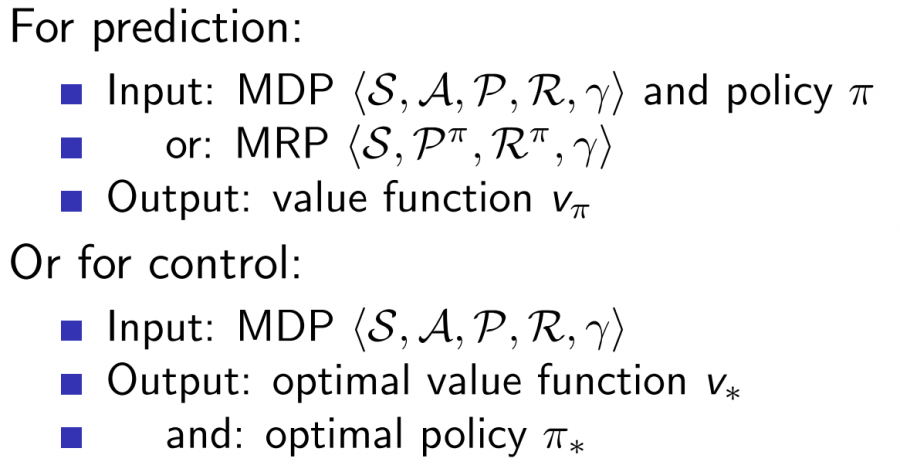## 2. 策略迭代 Policy Iteration

### 2.1 方法简介

• Policy Evaluation:基于当前的Policy计算出每个状态的value function $V$（迭代计算直到收敛）。
• Policy Improvment:基于当前的value function，采用贪心算法来找到当前最优的Policy $\pi$。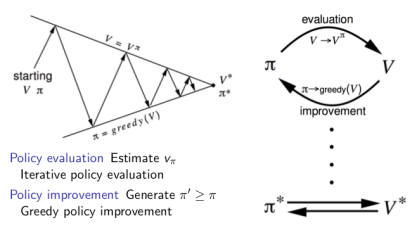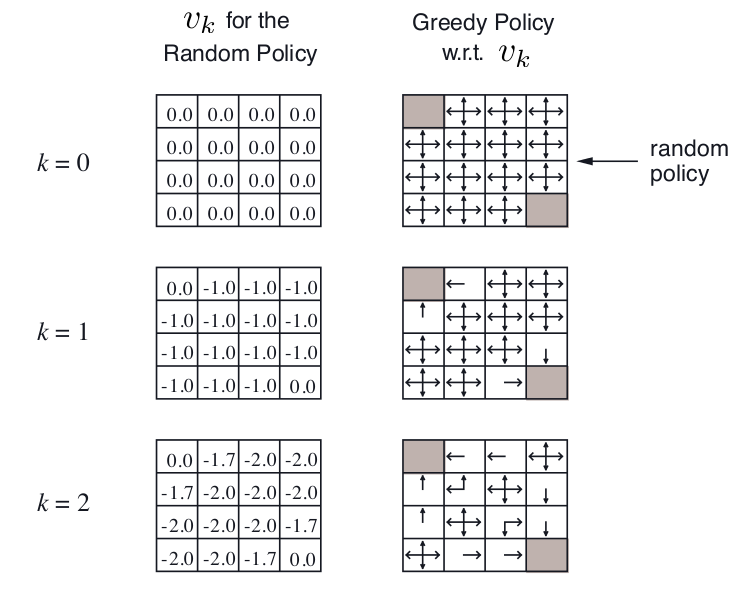### 2.2 方法证明

#### 2.2.1 Policy Evaluation收敛性证明

##### 2.2.1.2 压缩映射定理

• 在该度量空间中，存在唯一的点$x_{*}$满足$f\left(x_{*}\right)=x_{*}$。
• 并且，对于任意的$x \in M$, 定义序列$f^{2}(x)=f(f(x)), f^{3}(x)=f\left(f^{2}(x)\right) |, \cdots,f^{n}(x)=f\left(f^{n-1}(x)\right)$，该序列会收敛于$x_{*}$，即$\lim_{n \rightarrow \infty} f^{n}(x)=x_{*}$

##### 2.2.1.3 贝尔曼期望方程及其向量形式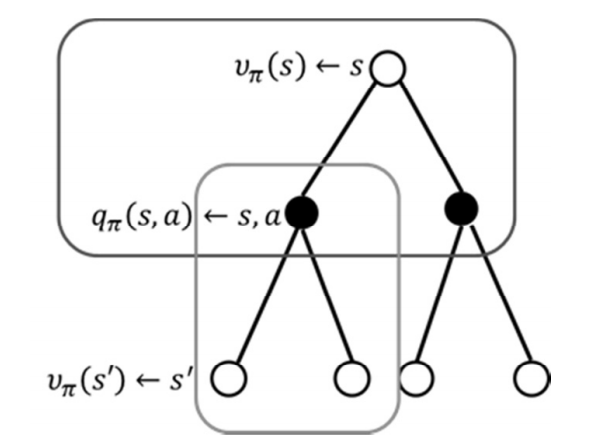$S=\left\{S_{0}, S_{1}, \ldots, S_{n}\right\}$

$V_{\pi}=\left\{V_{\pi}\left(s_{0}\right), V_{\pi}\left(s_{1}\right), \cdots, V_{\pi}\left(s_{n}\right)\right\}^{T}$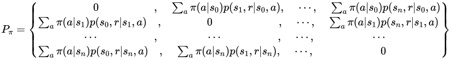$R_{\pi}=\left\{R_{0}, R_{1}, \cdots, R_{n}\right\}^{T}$

$V_{\pi}=R_{\pi}+\gamma P_{\pi} V_{\pi} \tag3$
##### 2.2.1.4 收敛性证明

$T_{\pi}(v)=R_{\pi}+\gamma P_{\pi} v$

\begin{equation} \begin{aligned} &\rho\left(T_{\pi}(u), T_{\pi}(v)\right) =\left|T_{\pi}(u)-T_{\pi}(v)\right|_{\infty} \\ &=\left|\left(R_{\pi}+\gamma P_{\pi} u\right)-\left(R_{\pi}+\gamma P_{\pi} v\right)\right|_{\infty} \\ &=\left|\gamma P_{\pi}(u-v)\right|_{\infty} \\ & \leqslant\left|\gamma P_{\pi}\right| u-v\left|_{\infty}\right|_{\infty} \\ & \leqslant \gamma\|u-v\|_{\infty} \end{aligned} \end{equation}

#### 2.2.2 Policy Improvement收敛性和最优性证明

$\pi^{\prime}(s)=\underset{a \in \mathcal{A}}{\operatorname{argmax}} q_{\pi}(s, a)$

$q_{\pi}\left(s, \pi^{\prime}(s)\right)=\max_{a \in \mathcal{A}} q_{\pi}(s, a) \geq q_{\pi}(s, \pi(s))=v_{\pi}(s)$

\begin{aligned} v_{\pi}(s) & \leq q_{\pi}\left(s, \pi^{\prime}(s)\right)=\mathbb{E}\left[R_{t+1}+\gamma v_{\pi}\left(S_{t+1}\right) | S_{t}=s,A_{t}=\pi'(s)\right] \\\ & = \mathbb{E}_{\pi^{\prime}}\left[R_{t+1}+\gamma v_{\pi}\left(S_{t+1}\right) | S_{t}=s\right] \\\ & \leq \mathbb{E}_{\pi^{\prime}}\left[R_{t+1}+\gamma q_{\pi}\left(S_{t+1}, \pi^{\prime}\left(S_{t+1}\right)\right) | S_{t}=s\right] \\\ & \leq \mathbb{E}_{\pi^{\prime}}\left[R_{t+1}+\gamma R_{t+2}+\gamma^{2} q_{\pi}\left(S_{t+2}, \pi^{\prime}\left(S_{t+2}\right)\right) | S_{t}=s\right] \\\ & \leq \mathbb{E}_{\pi^{\prime}}\left[R_{t+1}+\gamma R_{t+2}+\ldots | S_{t}=s\right]=v_{\pi^{\prime}}(s) \end{aligned}

$v_{\pi}(s)=\max_{a \in \mathcal{A}} q_{\pi}(s, a)$

$\pi(a | s)=\left\{\begin{array}{ll}{\epsilon / m+1-\epsilon} & {\text { if } a^{*}=\underset{a \in \mathcal{A}}{\operatorname{argmax}} Q(s, a)} \\ {\epsilon / m} & {\text { otherwise }}\end{array}\right.$

\begin{aligned} q_{\pi}\left(s, \pi^{\prime}(s)\right) &=\sum_{a \in \mathcal{A}} \pi^{\prime}(a | s) q_{\pi}(s, a) \\\ &=\epsilon / m \sum q_{\pi}(s, a)+(1-\epsilon) \max_{a \in \mathcal{A}} q_{\pi}(s, a) \\\ & \geq \epsilon / m \sum_{a \in \mathcal{A}} q_{\pi}(s, a)+(1-\epsilon) \sum_{a \in \mathcal{A}} \frac{\pi(a | s)-\epsilon / m}{1-\epsilon} q_{\pi}(s, a) \\\ &=\sum_{a \in \mathcal{A}} \pi(a | s) q_{\pi}(s, a)=v_{\pi}(s) \end{aligned}

## 3. 值迭代 Value Iteration

### 3.1 优化原则 Principle of Optimality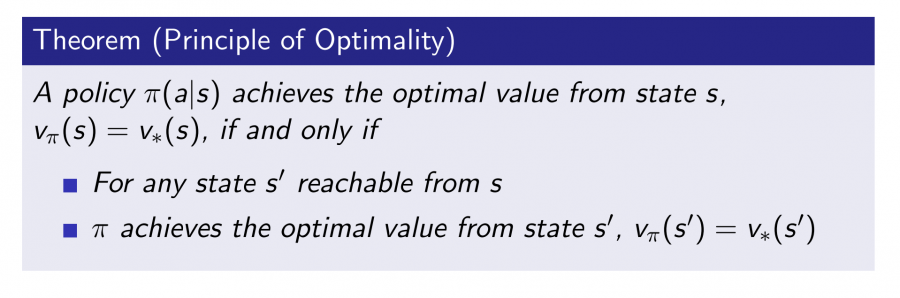### 3.2 方法简介

$v_{*}(s) \leftarrow \max _{a \in \mathcal{A}} \mathcal{R}_{s}^{*}+\gamma \sum_{s^{\prime} \in \mathcal{S}} \mathcal{P}_{\mathrm{ss}^{\prime}}^{4} v_{*}\left(s^{\prime}\right)$

Value Iteration通过迭代的方法，通过这一步的$v_{k}(s’)$更新下一步的$v_{k+1}(s)$，最终收敛到最优的$v_{∗}$，需要注意的是中间生成的value function的值不对应着任何policy。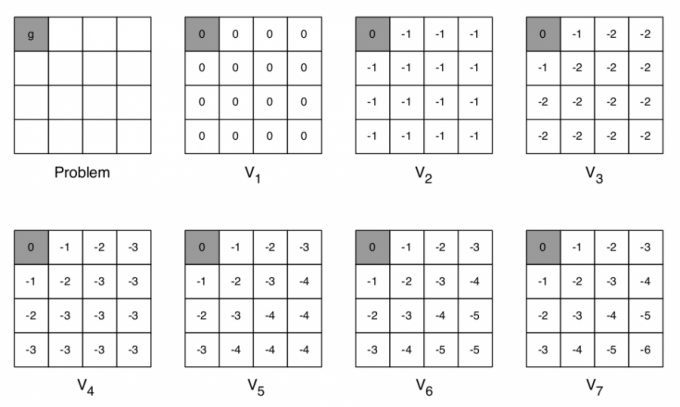## 4. PI与VI的缺陷

• 无法处理连续状态与动作空间
• 当状态空间巨大时存在维度诅咒的问题
• 不适用与POMDP
• 使用动态规划的方式要求知道状态转移概率与奖励，但在实际中往往无法获得

## 5. 总结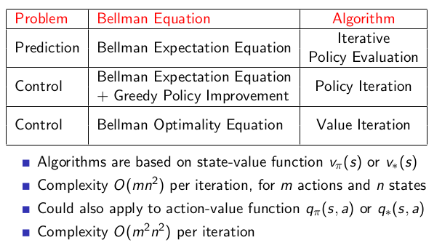##### Share